# 方差分析

• A+

### 单因素方差分析

(1)每一总体均为正态总体，记为N(μi , σi^2),i=1,2,...,k

(2)各总体的方差相同，记为σ1^2=σ2^2=...=σr^2=σ^2

(3)从每一总体中抽取的样本是相互独立的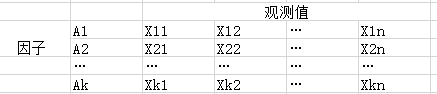1.提出假设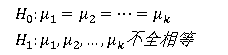2.构造检验统计量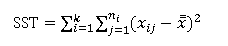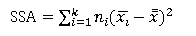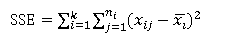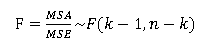3.统计决策

mathematica实现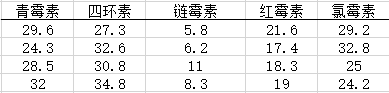``````Needs["ANOVA`"];(*使用发方差分析需要把此句写在前面*)
ANOVA[{{1, 29.6}, {1, 24.3}, {1, 28.5}, {1, 32.0},
{2, 27.3}, {2, 32.6}, {2, 30.8}, {2, 34.8},
{3, 5.8}, {3, 6.2}, {3, 11.0}, {3, 8.3},
{4, 21.6}, {4, 17.4}, {4, 18.3}, {4, 19.0},
{5, 29.2}, {5, 32.8}, {5, 25.0}, {5, 24.2}}]
(*每个括号内的数据填写方法是先写第几个因子，再顺序写下此因子下的观测值*)``````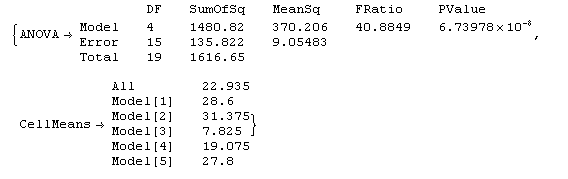### 双因素方差分析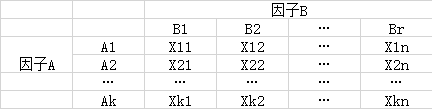1.提出假设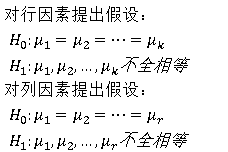2.构造检验统计量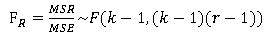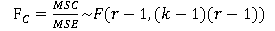3.统计决策

mathematica实现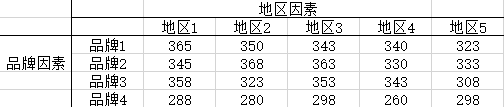``````Needs["ANOVA`"];
ANOVA[{{1, 1, 365}, {1, 2, 350}, {1, 3, 343}, {1, 4, 340}, {1, 5, 323},
{2, 1, 345}, {2, 2, 368}, {2, 3, 363}, {2, 4, 330}, {2, 5, 333},
{3, 1, 358}, {3, 2, 323}, {3, 3, 353}, {3, 4, 343}, {3, 5, 308},
{4, 1, 288}, {4, 2, 280}, {4, 3, 298}, {4, 4, 260}, {4, 5,
298}}, {x, y}, {x, y}]
(*每个括号内的数据填写方法是先写第几个行因子，第几个列因子，再写下此定位下的观测值*)``````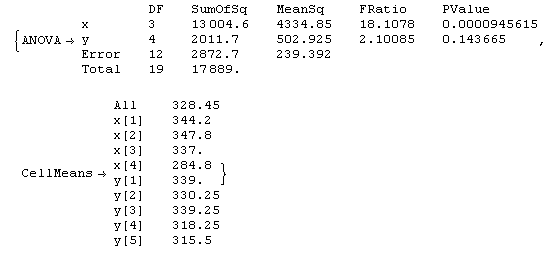• 微信公众号
• 关注微信公众号
•• QQ群
• 我们的QQ群号
•